SPSS TUTORIALS BASICS ANOVA REGRESSION FACTOR CORRELATION

# Which Statistical Test Should I Use?

## Summary

Finding the appropriate statistical test is easy if you're aware of

1. the basic type of test you're looking for and
2. the measurement levels of the variables involved.

For each type and measurement level, this tutorial immediately points out the right statistical test. We'll also briefly define the 6 basic types of tests and illustrate them with simple examples.

## 1. Overview Univariate Tests

MEASUREMENT LEVELNULL HYPOTHESISTEST
DichotomousPopulation proportion = x?Binomial test
Z-test for 1 proportion
CategoricalPopulation distribution = f(x)?Chi-square goodness-of-fit test
QuantitativePopulation mean = x?One-sample t-test
Population median = x?Sign test for 1 median
Population distribution = f(x)?Kolmogorov-Smirnov test
Shapiro-Wilk test

## Univariate Tests - Quick Definition

Univariate tests are tests that involve only 1 variable. Univariate tests either test if

1. some population parameter -usually a mean or median- is equal to some hypothesized value or
2. some population distribution is equal to some function, often the normal distribution.

A textbook example is a one sample t-test: it tests if a population mean -a parameter- is equal to some value x. This test involves only 1 variable (even if there's many more in your data file).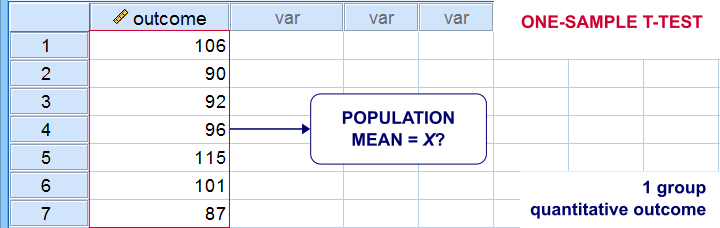## 2. Overview Within-Subjects Tests

MEASUREMENT LEVEL2 VARIABLES3+ VARIABLES
DICHOTOMOUSMcNemar test
Z-test for dependent proportions
Cochran Q test
NOMINALMarginal homogeneity test(Not available)
ORDINALWilcoxon signed-ranks test
Sign test for 2 related medians
Friedman test
QUANTITATIVEPaired samples t-testRepeated measures ANOVA

## Within-Subjects Tests - Quick Definition

Within-subjects tests compare 2+ variables
measured on the same subjects (often people).
An example is repeated measures ANOVA: it tests if 3+ variables measured on the same subjects have equal population means.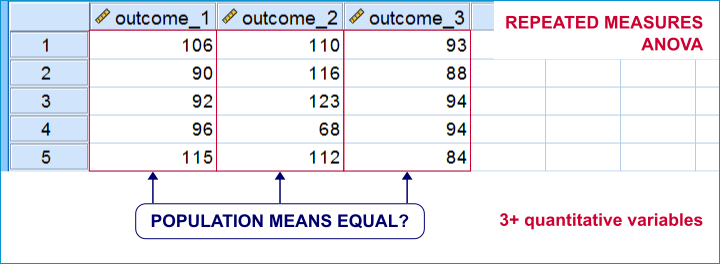Within-subjects tests are also known as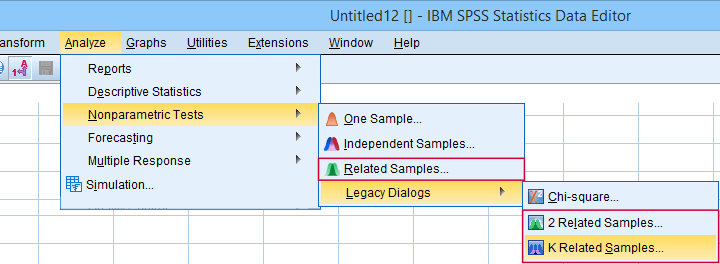“Related samples” refers to within-subjects and “K” means 3+.

## 3. Overview Between-Subjects Tests

OUTCOME VARIABLE2 SUBPOPULATIONS3+ SUBPOPULATIONS
DichotomousZ-test for 2 independent proportionsChi-square independence test
NominalChi-square independence testChi-square independence test
OrdinalMann-Whitney test (mean ranks)
Median test for 2+ independent medians
Kruskal-Wallis test (mean ranks)
Median test for 2+ independent medians
QuantitativeIndependent samples t-test (means)
Levene's test (variances)
One-way ANOVA (means)
Levene's test (variances)

## Between-Subjects Tests - Quick Definition

Between-subjects tests examine if 2+ subpopulations
are identical with regard to

• a parameter (population mean, standard deviation or proportion) or
• a distribution.

The best known example is a one-way ANOVA as illustrated below. Note that the subpopulations are represented by subsamples -groups of observations indicated by some categorical variable.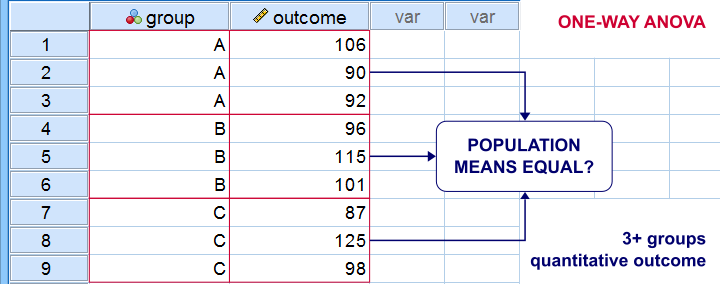“Between-subjects” tests are also known as “independent samples” tests, such as the independent samples t-test. “Independent samples” means that subsamples don't overlap: each observation belongs to only 1 subsample.

## 4. Overview Association Measures

(VARIABLES ARE) QUANTITATIVEORDINALNOMINALDICHOTOMOUS
QUANTITATIVEPearson correlation
ORDINALSpearman correlation
Kendall’s tau
Polychoric correlation
Spearman correlation
Kendall’s tau
Polychoric correlation
NOMINALEta squaredCramér’s V Cramér’s V
DICHOTOMOUSPoint-biserial correlation
Biserial correlation
Spearman correlation
Kendall’s tau
Polychoric correlation
Cramér’s V Phi-coefficient
Tetrachoric correlation

## Association Measures - Quick Definition

Association measures are numbers that indicate
to what extent 2 variables are associated.
The best known association measure is the Pearson correlation: a number that tells us to what extent 2 quantitative variables are linearly related. The illustration below visualizes correlations as scatterplots.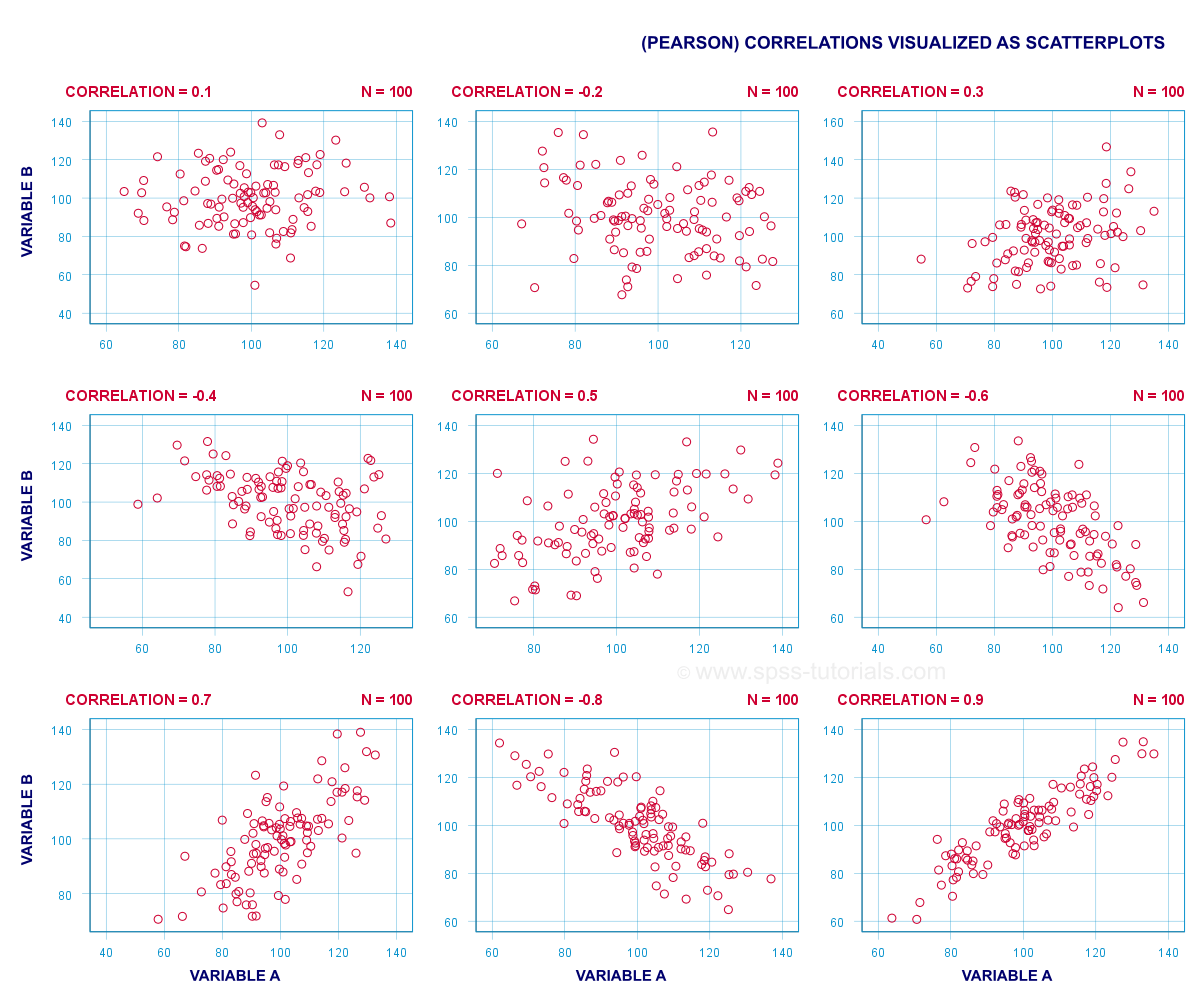## 5. Overview Prediction Analyses

OUTCOME VARIABLEANALYSIS
Quantitative(Multiple) linear regression analysis
OrdinalDiscriminant analysis or ordinal regression analysis
NominalDiscriminant analysis or nominal regression analysis
DichotomousLogistic regression

## Prediction Analyses - Quick Definition

Prediction tests examine how and to what extent
a variable can be predicted from 1+ other variables.
The simplest example is simple linear regression as illustrated below.Prediction analyses sometimes quietly assume causality: whatever predicts some variable is often thought to affect this variable. Depending on the contents of an analysis, causality may or may not be plausible. Keep in mind, however, that the analyses listed below don't prove causality.

## 6. Classification Analyses

Classification analyses attempt to identify and
describe groups of observations or variables.
The 2 main types of classification analysis are

• factor analysis for finding groups of variables (“factors”) and
• cluster analysis for finding groups of observations (“clusters”).

Factor analysis is based on correlations or covariances. Groups of variables that correlate strongly are assumed to measure similar underlying factors -sometimes called “constructs”. The basic idea is illustrated below.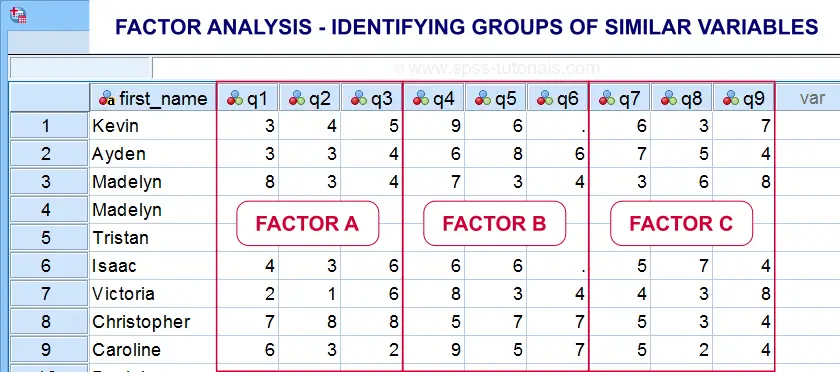Cluster analysis is based on distances among observations -often people. Groups of observations with small distances among them are assumed to represent clusters such as market segments.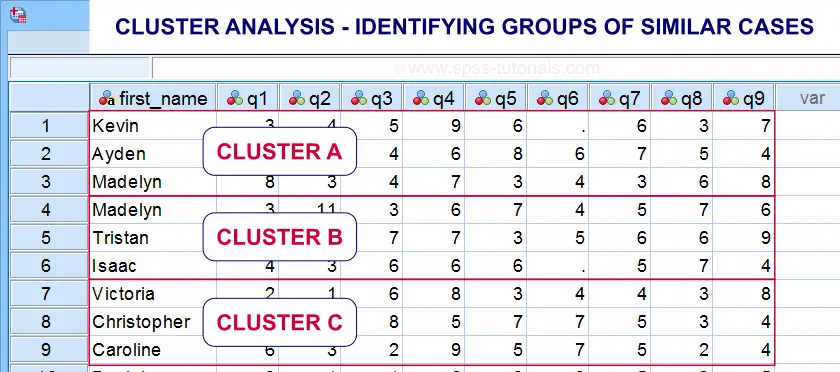Right. So that'll do for a basic overview. Hope you found this guide helpful! And last but not least,

# Tell us what you think!

*Required field. Your comment will show up after approval from a moderator.

# THIS TUTORIAL HAS 15 COMMENTS:

•### By N M on May 18th, 2021

Hi could you do a post on point biserial and biserial please ? Thank you so much for the work you do

•### By Maxine Duncan on July 6th, 2022

This article was a valuable source of information to me.

•### By Roger ILUNGA NTABALA on July 18th, 2022

Verry good

•### By YY on July 19th, 2022

A comprehensive guide. Very useful!
Can the McNemar-Bowker test provided by SPSS be used when there are two related nominal variables?

•### By Ruben Geert van den Berg on July 20th, 2022

Yes. We haven't examined this procedure very thoroughly but I believe that's correct.